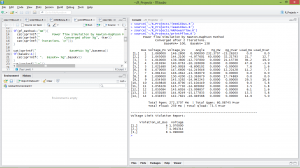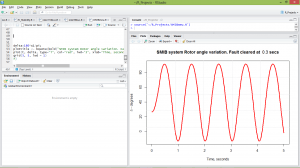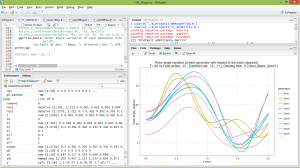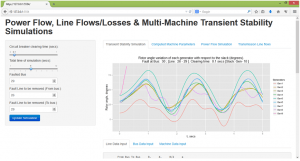This is a guest post by Ben Ubah.

The field of electric power systems engineering relies heavily on computer simulations for analysis because of its nature. These computer simulations aid the planning, operation and management of the system. Computer simulations have been implemented using several scientific computing tools. However, I have not yet seen any implementations using R. This inspired my thesis at a German institution. I am now privileged to have implemented several power systems analysis simulations using R. In the process, I started by creating standalone scripts for several topics of interests in this domain. Examples are:

• Power flow simulation (Gauss-Siedel & Newton-Raphson techniques)
• Time domain simulation of the Single-Machine Infinite Bus (SMIB) system using the Modified Euler numerical integration method.
• Multi-machine transient stability simulation using the ‘deSolve’ package for solving n-ODEs that describe the dynamics of interconnected generating machines.
See screenshots below.Figure 1: Power flow simulation of the IEEE 14-bus system (Newton-Raphson technique) The power flow simulation consists of evaluating the flow of power and voltages of a network for a specified terminal or bus condition. The results of a power flow solution are used to evaluate loadability of lines or transformers and the acceptability of voltage profiles.Figure 2: Time domain simulation of an SMIB system The infinite bus has a great advantage in explaining many of the problems associated with power systems. When analytical solutions are sought, the infinite busbar provides a reference for all angular changes in power system parameters. This simulation concerns the transient stability performance of a single generator that is connected to the infinite bus (or grid).Figure 3: Transient stability simulation of the IEEE 39-bus 10 generator system Transient stability analysis, deals with the effects of large, sudden disturbances such as the sudden outage of a line, the occurrence of a fault or the sudden loss of load. Studying the transient stability of the power system is required to understand whether the system can withstand the transient situation following a major perturbation. It is very insightful in the planning and operation of power systems. I also went ahead to try out web-based implementations for these simulations and the results were great. The ‘shiny‘ package developed by RStudio was used to implement this (interactivity through reactive programming was achieved). The use of tabpanels provided an integration of many simulation views (e.g. one can view power flow and stability simulations on one web page). The reactive programming concept solved the problem of always going back to the input data file to modify some system parameters before re-simulation. For example, in the web-based multi-machine transient stability simulation program, the circuit breaker clearing time, time of simulation, faulted bus and line to be cleared are reactive. See screenshot (of Figure 4) below:Figure 4: Web-based Power flow and Multi-machine transient stability simulation These few simulations that were used as a case study for my thesis, have since then grown into ‘The RPowerLABS Project‘ with diverse simulations and potentials. In a subsequent post, I shall highlight the offerings of this project and its impact for developing nations. Some reasons why R is suitable for electrical power system engineering analysis and simulation are:
• R can handle complex number operations
• R is great at basic mathematical operations
• Vector/Matrix arithmetic operations are very possible with R
• Numerical linear algebra are well taken care of
• Sparse matrices are catered for by R (or addon packages)
• R has very attractive visualization provisions
If R is proven suitable for electrical power system engineering analysis, how about other engineering sub-domains?   Note: The major idea of this post is to inform the R community of the application of R to electric power system engineering and there exists a lot of potential for this in the academia (especially e-learning). Hence, we have not shown the nitty gritty of the simulation examples in this post. Watch out for other related posts upcoming!